# C Program to check sum is less than or greater than hundred

C Program to check sum is less than or greater than hundred
Input :-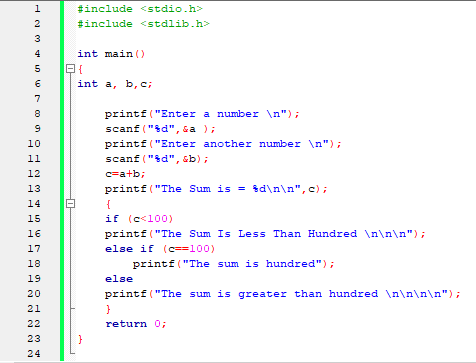Output :-
1)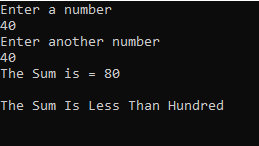2)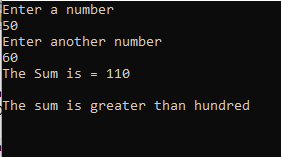3)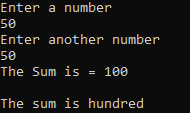Code :-

#include <stdio.h>
#include <stdlib.h>

int main()
{
int a, b,c;

printf("Enter a number \n");
scanf("%d",&a );
printf("Enter another number \n");
scanf("%d",&b);
c=a+b;
printf("The Sum is = %d\n\n",c);
{
if (c<100)
printf("The Sum Is Less Than Hundred \n\n\n");
else if (c==100)
printf("The sum is hundred");
else
printf("The sum is greater than hundred \n\n\n\n");
}
return 0;
}

# Lecture 53 Preincrement , PostIncrement Operators in C

Preincrement  , PostIncrement  Predecrement  , Postdecrement Operators in C

# Lecture 52 The C preprocessor

The C Preprocessor

# Lecture 51 Practices for writing larger programs in C

# Lecture 48 Pointers to Structures

# Lecture 47 Structures in C

Structures in C

# Lecture 46 Some other file handling functions

Some other file handling functions

# Lecture 44 Multidimensional arrays and pointers continued

Multidimensional arrays and pointers continued

# Lecture 41 Multidimensional Arrays

Multidimensional Arrays

# Lecture 40 Recursion Two way Recursion

Recursion Two way Recursion

# Lecture 39 Recursion Linear recursion 2

Recursion Linear recursion 2

# Lecture 38 Recursion Linear recursion

Recursion Linear recursion

# Lecture 37 Example for returning pointers return the duplicate of a string

Example for returning pointers return the duplicate of a string

# Lecture 36 Returning pointers from functions

Returning pointers from functions

# Lecture 35 Size of operator how pointer arithmetic works

Size of operator how pointer arithmetic works

# Lecture 32 Function with pointer arguments

Function with pointer arguments

# Lecture 29 Initializing character arrays

Initializing character arrays

# Lec 28 Initializing arrays

Initializing arrays

# Lecture 26 Some examples on functions

Some examples on functions

# Lecture 25 Some examples on functions

Some examples on functions

# Lecture 24 How functions are executed

How functions are executed

# Lecture 22 Expression evaluation mod01

Expression evaluation

# Lecture 21 Precedence of C operators

Precedence of C operators

# Lecture 20 C operators and expressions associativity of operators

C operators and expressions associativity of operators

# Lecture 19 The break statement

The break statement

# Lecture 18 A matrix problem using nested for loops

A matrix problem using nested for loops

Data types in C

# Lecture 14 A matrix problem nested loops

A matrix problem nested loops

# Lecture 11 More while loops Longest Increasing Subsequence 2

More while loops Longest Increasing Subsequence 2

# Lecture 10 More while loops Longest Increasing Subsequence 1

More while loops Longest Increasing Sub sequence 1

# C Program for Addition of Two Numbers

Program for addition of two numbers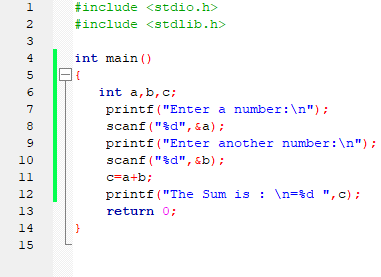Output: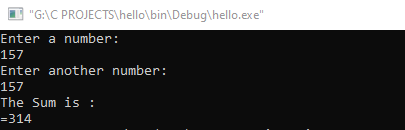Code :-

#include <stdio.h>
#include <stdlib.h>

int main()
{
int a,b,c;
printf("Enter a number:\n");
scanf("%d",&a);
printf("Enter another number:\n");
scanf("%d",&b);
c=a+b;
printf("The Sum is : \n=%d ",c);
return 0;
}

# Lecture 9 - While Loop Example GCD

While Loop Example GCD

# Lecture 8 - While Loop Example

While Loop Example

# Lecture 7 - While Loop 1

While Loop Example
In this lecture we will learn about introduction to while loop.

# Lecture 6 :- Operators in C

Operators in C
In this lecture we will learn about different types of operators in C.

# Lecture 5 :- Variables in C

Variables in C
In this lecture we will learn about variables in C

# Lecture 4 Tracing a Simple Program

Tracing a Simple Program

In this video we will learn  how to write a basic program in C.How to run a program in C.

# The Programming Cycle

In this video we will learn about what are the basics steps to run a program in C

# Lecture 2: Introduction to Greatest Common Divisor

In this video you will learn about Euclid's Greatest Common Divisor (Theorem) and its relation with C language.We will also learn some basics step to run a program.

# Introduction The Process of Programming

## Categories

Android (21) AngularJS (1) Books (10) C (75) C# (4) C++ (81) Course (1) Downloads (1) Engineering (13) FPL (17) Hadoop (1) HTML&CSS (38) IS (25) Java (87) Leet Code (4) PHP (20) Projects (1) Python (218) R (69) Software (14) SQL (27)